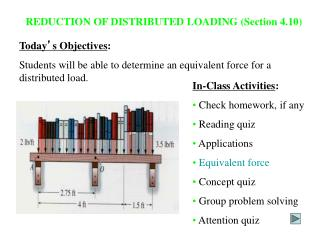DownloadDownload PresentationTélécharger la présentation- - - - - - - - - - - - - - - - - - - - - - - - - - - E N D - - - - - - - - - - - - - - - - - - - - - - - - - - -
##### Presentation Transcript

1. REDUCTION OF DISTRIBUTED LOADING (Section 4.10) Today’s Objectives: Students will be able to determine an equivalent force for a distributed load. • In-Class Activities: • Check homework, if any • Reading quiz • Applications • Equivalent force • Concept quiz • Group problem solving • Attention quiz

2. READING QUIZ Distributed load curve w 1. The resultant force (FR) due to a distributed load is equivalent to the _____ under the distributed loading curve, w = w(x). A) centroid B) arc length C) area D) volume x F R 2. The line of action of the distributed load’s equivalent force passes through the ______ of the distributed load. A) centroid B) mid-point C) left edge D) right edge

3. APPLICATIONS A distributed load on the beam exists due to the weight of the lumber. Is it possible to reduce this force system to a single force that will have the same external effect? If yes, how?

4. APPLICATIONS (continued) The sandbags on the beam create a distributed load. How can we determine a single equivalent resultant force and its location?

5. DISTRIBUTED LOADING In many situations a surface area of a body is subjected to a distributed load. Such forces are caused by winds, fluids, or the weight of items on the body’s surface. We will analyze the most common case of a distributed pressure loading. This is a uniform load along one axis of a flat rectangular body. In such cases, w is a function of x and has units of force per length.

6. MAGNITUDE OF RESULTANT FORCE Consider an element of length dx. The force magnitude dF acting on it is given as dF = w(x) dx The net force on the beam is given by +  FR = L dF = L w(x) dx = A Here A is the area under the loading curve w(x).

7. The total moment about point O is given as + MRO = L x dF = L x w(x) dx Assuming that FR acts at , it will produce the moment about point O as + MRO = ( ) (FR) = L w(x) dx LOCATION OF THE RESULTANT FORCE The force dF will produce a moment of (x)(dF) about point O.

8. LOCATION OF THE RESULTANT FORCE (continued) Comparing the last two equations, we get You will learn later that FR acts through a point “C,” which is called the geometric center or centroid of the area under the loading curve w(x).